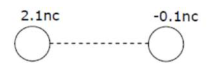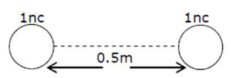# Two identical conducting spheres with negligible volume have 2.1 nC and -0.1 nC charges,

Question:

Two identical conducting spheres with negligible volume have $2.1 \mathrm{nC}$ and $-0.1 \mathrm{nC}$ charges, respectively. They are brought into contact and

then separated by a distance of $0.5 \mathrm{~m}$. The electrostatic force acting between the spheres is $\times 10^{-9} \mathrm{~N}$

[Given : $4 \pi \varepsilon_{0}=\frac{1}{9 \times 10^{9}}$ SI unit]

Solution:When they are brought into contact \& then separated by a distance $=0.5 \mathrm{~m}$

Then charge distribution will beThe electrostatic force acting $\mathbf{b} / \mathbf{w}$ the sphere is

$\mathrm{F}_{\mathrm{e}}=\frac{\mathrm{kq}_{1} \mathrm{q}_{2}}{\mathrm{r}^{2}}$

$=\frac{9 \times 10^{9} \times 1 \times 10^{-9} \times 1 \times 10^{-9}}{(0.5)^{2}}$

$=\frac{900}{25} \times 10^{-9}$

$F_{e}=36 \times 10^{-9} \mathrm{~N}$